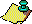Contents Next: References Up: A probabilistic model Previous: Consequences of the

Now, let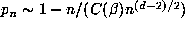be the probability that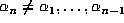. Then the probability that the points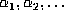are distinct is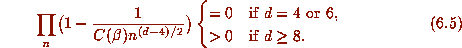This suggests that almost all orbits are finite if n = 4,6 while a positive proportion are infinite if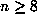.

We can distinguish the cases n = 4 and n = 6 by considering the expected size (D = m+p) of the orbit. Using the familiar fact that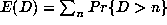, we compute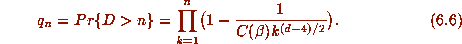For d =4, we thus have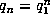, so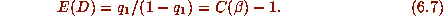On the other hand, if d = 6, then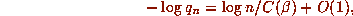i.e.,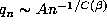, so that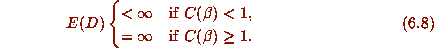Remark 6.2 For d = 4, we have shown rigorously in  that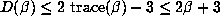. The largest value of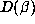for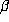with a fixed value of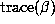is attained by thewith minimal polynomial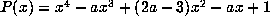. By (2.4), P has discriminant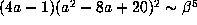for large, so that from (6.4),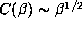. Thus (6.7) predicts a smaller value forthan actually attained but it does predict that large values ofoccur for small values of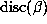and vice versa.

Remark 6.3 For d = 6, the data tends to confirm a direct relationship between the sizes of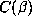and, although not quite as dramatic as (6.8) would suggest. There are some exceptions as well: for example if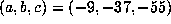, then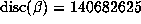is quite small, so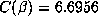is large, but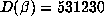is not exceptionally large. On the other hand, the example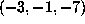with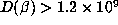only has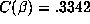, which is not very large (although it is the largest value ofby far in Table 3, i.e., for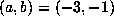). This is perhaps to be expected, given the number of unsupported assumptions we have had to make to arrive at (6.8).

Perhaps the most questionable argument is the deduction of the exponent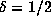in section 6.4. Let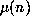denote the maximum modulus of the conjugates of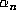, i.e., the largest modulus of the eigenvalues of the matrix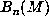discussed in section 5.2. A fit of a power curve to data from the first 20000 values offor the case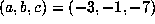suggests an exponent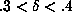for this example. A plot ofversus n in this range suggests some sort of random process but perhaps not one well described by the model of section 6.4. Note that, for d = 6, we only need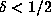to apply the birthday paradox argument of section 6.3, so if the growth observed for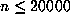were to persist, then section 6.3 would predict a periodic beta expansion for this.

To test this, let us consider the behavior of the record values of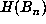for large n still for this. So, let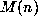denote the largest value of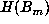for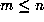. For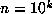,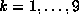, we find that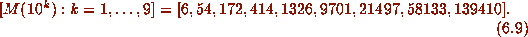Fitting a power curve to these 9 values (by a linear least squares fit to their logarithms) gives a curve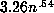, suggesting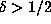. On the other hand, using only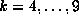gives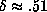, while the data for the 5 values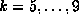is very well fitted by a curve with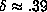. Apparently, this data does not exclude or confirm a growth rate of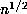. Another instructive example is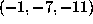, where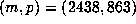. Here,is quite well fitted for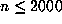by a curve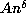with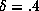. Of course, this cannot hold forsinceis ultimately periodic.

Remark 6.4 Our computations have concentrated on the question of whether Salem numbers are beta numbers and not on Schmidt's more general conjecture that, for every Salem numberand every rational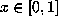, the orbit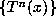should be finite. Our heuristic arguments apply equally well to this conjecture. If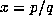, then the iterates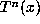lie in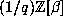, and hence we need only replace the lattice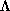by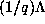in the arguments of section 6.1 to section 6.6. The only change is that the density of the points ofin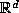is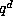times as large as the density of the points of. Thus, the qualitative predictions of section 6.1 to section 6.4 are unchanged, i.e., we predict that Schmidt's conjecture is true for Salem numbers of degree 4 and 6 but not for higher degrees. The main change in the more quantitative analysis of section 6.5 and section 6.6 is thatmust be replaced by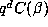. Thus, section 6.6 would suggest that, for fixed, the size of the orbit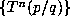should increase with q as predicted by (6.7) and (6.8). That is, for d = 4 the size of the orbit should be roughly proportional to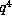, and for d = 6 we should expect unusually large orbits as soon as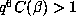. It would be interesting to test these predictions by further computation.Contents Next: References Up: A probabilistic model Previous: Consequences of the